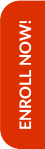Olympiads are the stepping stones to achieve better results in the competitive world that lies ahead in the life of the child. Math Olympiad examinations help students to improve their mathematical skills along with their analytical and problem solving abilities. Along with that CBSE syllabus also plays a crucial role in the same.

Hence, Olympiad Success Live has designed a long-term course for Mathematics preparation which includes both CBSE syllabus and Olympiad for class 8. This long-term course will help in building the foundation of the child. For this, we have done great efforts in finding the tutor for class 8 Math CBSE and Olympiad preparation with relevant background and experience.

If you are interested in purchasing this course, then please Enrol Now. You will be redirected to the batch detail page, wherein you can see all the details like batch start and demo dates, fess and the registration link related to Math CBSE and Olympiad for class 8 course.

Grade 8 CBSE Mathematics syllabus identifies age-appropriate content. During the learning of Mathematics from grade 6 to grade 8, a child:

• Moves from number sense to number patterns;
• Sees relationships between numbers and looks for patterns in relationships;
• Gains proficiency in using the newer language of Mathematics like variables, expressions, equations, identities, etc.
• Uses arithmetic and algebra to solve real-life problems and pose meaning problems;
• Discovers symmetries and acquire a sense of aesthetics by looking around regular shapes like triangles, circles, quadrilaterals, etc;
• Comprehends the idea of space as reason enclosed within boundaries of a shape;
• Relates numbers with shapes in terms of perimeter, area and volume and uses them to solve everyday life problems;
• Learns to provide reasoning and convincing arguments to justify her/his own conclusions particularly in Mathematics; and
• Collects, represents (graphically and in tables) and interprets data/information from her/his life experiences

The content of grade 8 CBSE Maths is consistent with the development of the student which includes:

• Number System
• Algebra
• Ratio and Proportion
• Geometry
• Representing 3-D in 2-D
• Mensuration
• Data Handling

Check the detailed syllabus below. For Olympiads preparation, you can check the syllabus here!

Keeping in mind the curricular expectations, Olympiad Success Live has designed a course for CBSE and Olympiads Maths preparation for class 8 students. And for this, we have the right set of tutors with relevant backgrounds and experience for class 8 Maths CBSE and Olympiads preparation.

If you are interested in enrolling in this grade 8 CBSE and Olympiads Math course, then you can pay the fees here. After payment, kindly WhatsApp us your name, class and subject at +91 95607 64447

##### Syllabus

Number System

• Rational Numbers
• Properties of rational numbers
• Pperations on rational numbers
• Represent rational numbers on the number line
• Between any two rational numbers, there lies another rational number
• Word problems using rational numbers
• Powers
• Laws of exponents with integral powers
• Square and square roots using factor method and division method for numbers containing
• no more than total 4 digits and
• no more than 2 decimal places
• Cubes and cube roots (only factor method for numbers containing at most 3 digits)
• Playing with numbers
• Writes and understands a two and three digit number in generalised form (100a + 10b + c, where a, b, c can be only digit 0-9) and engages with various puzzles.
• Solves and creates problems and puzzles.
• Deduces the divisibility test rules of 2, 3, 5, 9, 10 for a two or three-digit number expressed in the general form.

Algebra

• Algebraic Expressions
• Multiplication and division of algebraic expressions (Coefficient should be integers)
• Identities (a ± b)2 = a2± 2ab + b2, a2 – b2 = (a – b) (a + b)
• Factorise expressions
• Linear equations in one variable

Ratio and Proportion

• Applications on percentages, profit and loss, overhead expenses, discount, and taxes
• Simple and compound interest (compounded yearly up to 3 years or half-yearly up to 3 steps only)
• Direct and inverse variations
• Simple and direct word problems
• Time and work problems– Simple and direct

Geometry

• Shapes
• Properties of quadrilaterals – Angle sum property
• Properties of parallelogram (By verification)
• Opposite sides of a parallelogram are equal
• Opposite angles of a parallelogram are equal
• Diagonals of a parallelogram bisect each other
• Diagonals of a rectangle are equal and bisect each other
• Diagonals of a rhombus bisect each other at right angles
• Diagonals of a square are equal and bisect each other at right angles

Representing 3-D in 2-D

• Nested, joint 2-D and 3-D shapes
• 2-D representation of 3-D objects (Continued and extended)
• Euler’s relation for 3-D figures with flat faces (cubes, cuboids, tetrahedrons, prisms and pyramids)

• Four sides and one diagonal
• Three sides and two diagonals
• Three sides and two included angles
• Two adjacent sides and three angles

Mensuration

• Area of a trapezium and a polygon
• Surface area of a cube, cuboid, cylinder
• Volume, measurement of volume using a basic unit, volume of a cube, cuboid and cylinder
• Measurement of capacity

Data handling

• Representation of grouped data through bar-graphs, constructing and interpreting bar- graphs
• Simple pie charts with reasonable data numbers
• Notion of chance in events like tossing coins, dice, etc.

Online classes started for English, Mathematics, Science and Reasoning for classes (3rd to 10th)